## Redox ReactionsThe reaction in which oxidation and reduction both occur simultaneously is Redox reactions.

Let us understand about oxidation and reduction. We have different concepts to understand oxidation and reduction.

Chemical reactions that involve both the oxidations and the reduction process simultaneously are redox reactions (‘red’) from reduction and (‘ox’) from oxidation.

All these reactions are always accompanied by energy change in the form of heat, light or electricity.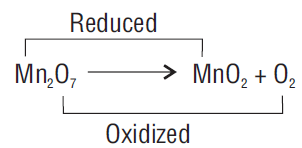• The processes of oxidation and reduction occur simultaneously and cannot happen independently of one another, similar to the acid-base reaction.
• The oxidation alone and the reduction alone are each called a half-reaction because two half-reactions always occur together to form a whole reaction.
• When writing half-reactions, the gained or lost electrons are typically included explicitly in order that the half-reaction is balanced with respect to electric charge.

Many important biological processes involve redox reactions.

### Oxidation and Reduction

In terms of Classical concept:
Oxidation: We define Oxidation as “addition of oxygen or any electronegative element and removal of hydrogen or an electropositive element” For example:

 Mg – 2e → Mg+2 Magnesium Magnesium ion

Reduction: We define Reduction as” addition of hydrogen or any electropositive element and removal of oxygen or any electronegative element”.

 Br2 + H2S → HBr + S CuO + H2 → Cu + H20

Please note the substance that undergoes oxidation itself and reduces other is a reducing agent whereas the substance that undergoes reduction itself and oxidizes other is an oxidizing agent.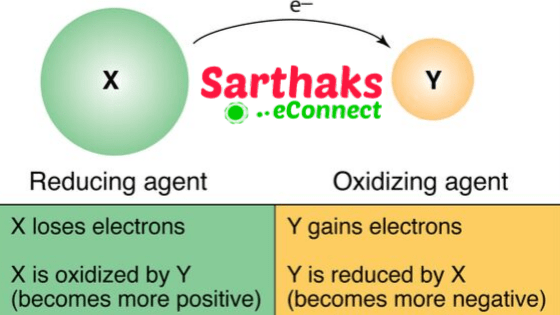In terms of Electron transfer concept:
Oxidation: Is defined as a loss of electrons that is:M- electron → M+ Metal → Metal

Reduction: Is defined as gain of electrons that is given below.

 N + electron → N- Non-metal Non-metal

Let’s consider equation:             H2    +   O2  →    H2O

• We can see in this example that H atom as going from neutral to a positive state in water, the O atom goes from zero states to a negative state in water. There is an electron transfer from Hydrogen to Oxygen and consequently, it oxidizes to Oxygen and it and reduces O2.
• The charge transfer is only partial or we can say that it is electron shift rather than calling it as complete loss of an electron by H and gain by O.
 Mg + H2 → MgH2 Magnesium Hydrogen Magnesium Hydride

In this Magnesium is losing electrons, that is undergoing oxidation and hydrogen is gaining electrons therefore, it is undergoing reduction. Mg is Reducing agent in it and H is Oxidizing agent in it.

## In terms of oxidation number:

• Oxidation number: We define it as “residual charge that is present on atom when the atom is in a combined state with other atoms”.
• We define the oxidation number as the charge in which an atom appears to have when all other atoms are removed from it as ions. It may have + or – sign.
• An element may have different values of oxidation number depending upon the nature of a compound in which it is present.
• The oxidation number of an element may be a whole number (positive or negative) or fractional or zero.

## Distinguish between Oxidation and Reduction Reaction:

 Oxidation Reduction Loss of electron. A gain of an electron. Addition of oxygen atom. Addition of hydrogen atom Removal of hydrogen and any electropositive metal Removal of oxygen and any electronegative metal

## Distinguish between Oxidizing and Reducing agent:

 Oxidizing agent Reducing agent The substance undergoes reduction itself and oxidizes other The substance undergoes oxidation itself and reduces other The strongest oxidizing agent is fluorine with the largest positive number for standard electrode potential lithium at the top of the list has the most negative number, indicating that it is the strongest reducing agent.

### Rules to assign and calculate the oxidation number:

• We take the oxidation number of atoms in their elemental state as zero.
• The oxidation number of mono-atomic atoms like Na+ etc is 1.
• And of Hydrogen is +1 when present with non-metals and -1 when present with metals.
• The oxidation number of oxygen is -2 in most of the compounds but in peroxides, it is -1.
The metals always have an oxidation number in positive and non-metal in negative when present together in ionic compounds.
• In compounds that have two atoms with different electronegativities, the oxidation number of more electronegative is taken as –ve and for less electronegative it is taken as positive. For example: In OF2 the oxidation number of oxygen will be in positive and oxidation of fluorine will be in negative.
• In neutral compounds, the sum of all oxidation numbers is equal to zero.
In complex ions, the sum of oxidation states of all the atoms is equal to the charge present on the complex.

#### Important Oxidation Number and State:

S in H2S2O8 : Here two O-atoms form peroxide linkage, i.e.,hence, oxidation no is +6

N in NH4NO3: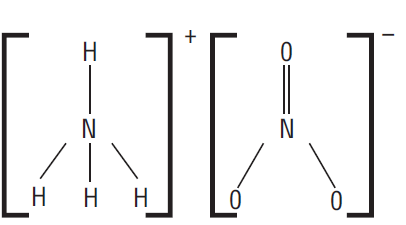Oxidation Number of N in NH4+ and Oxidation number of N in NO3

= -3      and    = +5

S in Na2S2O3: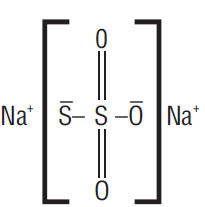The O.N. of S atom is –1 and of central S = + 5

But, here  Average oxidation no is +2

S in Na2S4O6: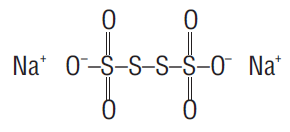hence, oxidation no is 2.5

Cr in CrO5: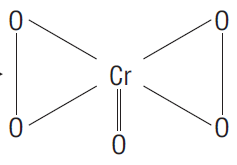hence, oxidation no is = 6

Oxidation: It is defined as an increase in oxidation number of an element.

 Mg + H2 → Mg2+H2 Magnesium Hydrogen MagnesiumHydride

Reduction: It is defined as a decrease in the oxidation number of an element.

 Fe3+ +   e- → Fe2+ Ferric Iron Ferrous Iron

In this oxidation number of iron is reduced. Therefore, the reduction occurs.

#### Redox reaction in terms of oxidation number:

• In this, Zinc is undergoing oxidation and act as reducing agent whereas, Hydrogen is undergoing reduction and act as an oxidizing agent.
 Zn0  +   HCl → Zn2+   +   H2O Zinc HydrogenChloride Zinc Chloride Hydrogen Gas

## Stock Notations:

• The oxidation states of elements exhibiting variable oxidation states are specified by Roman numerals such as I, II, III, IV, etc., within parenthesis after the symbol or name of the element.
• This system was introduced for the first time by a German chemist, Alfred Stock and is known as Stock notation. We may illustrate this as
 Formula Chemical name Stock Notation Cu2O Cuprous oxide Copper (I) oxide;Cu2(I)O Fe2O3 Ferric oxide Iron (III) oxide; Fe2(III)O3 HgCl2 Mercuric chloride Mercuric(II) chloride;Hg(III) Cl2 SnCl2 Stannous chloride Stannous(II) chloride;Sn(II) Cl2

## Balancing of Redox reactions:

There are two ways of balancing Redox reactions:

• Oxidation number method
• Half equation method

### Oxidation method:

• Write the skeletal equation of reactants and products.
Show the oxidation number of all the elements involved in the reaction.
• Calculate the increase or decrease in oxidation number per atom. Also, identify the oxidizing and reducing agents.
• Multiply the formula of oxidizing agent and reducing agent by suitable integers, to equalize the total increase or decrease in oxidation number as calculated in step c.

## Balancing of Redox reactions

• Balance all atoms other than H and O.
• Finally, balance H and O atoms by adding water molecules using hit and trial method.
• In the case of Ionic reactions:

#### For acidic medium

• First balance O atoms by adding water molecules to the deficient side.
• Balance H+ ions to the side deficient in H atoms.
##### For Basic medium
• First balance oxygen atom by adding water molecules to the deficient side.
• Then to balance hydrogen, add water molecules equal to the number of deficiency of H atoms.
• Also, add an equal number of OH- ions to the opposite side of the equation.
###### Half reaction method or Ion electron method:

Write the skeletal equation and Show the oxidation number of all the elements which occur in skeletal equation Find out the species that are oxidized and reduced. Split the skeletal equation into two half-reactions: oxidation half-reaction and reduction half reactionBalance the two-half equation separately by rules described below:

• In each half-reaction first balance the atoms of an element that has undergone a change in oxidation number.
• Add electrons to whatever side is necessary to make up the difference in oxidation number in each half-reaction.
• Balance the charge by adding H+ ions, if the reaction occurs in acidic medium. For basic medium, add OH ions if the reaction occurs in basic medium.
• Balance oxygen atoms by adding the required number of water molecules to the side deficient in oxygen atoms
• In the acidic medium, H atoms are balanced by adding H + ions to the side deficient in H atoms.
• However, in the basic medium H atoms are balanced by adding water molecules equal to the number to H atoms deficient.
• Add an equal number of OH- ions to the opposite side of the equation.
• The two half-reactions are then multiplied by suitable integers .so that the total number of electrons gained in half-reaction becomes equal to a total number of electrons lost in another half-reaction.
• Then the two half-reactions are added up.
• To verify the balancing, check whether the total charge on either is equal or not.

## Types of Redox reactions:

Combination reaction: When two or more reactants react to form a single product.

Decomposition reaction: It is the type of reaction, in which a reactant breaks up into its constituents either by heating, passing current or in presence of light.

Displacement reaction: It is the reaction between an element and a compound. In this, more reactive element displaces less reactive from its compound. Displacement reactions fit into two categories: metal displacement and non-metal displacement.

Metal displacement: a metal in a compound can be displaced by another metal in the uncombined state. Metal displacement reactions find many applications in metallurgy

Non-metal displacement: The non-metal displacement involves mainly the displacement of hydrogen and a rarely occurring reaction involving oxygen displacement.

Disproportionate reaction: A reaction in which the same species is simultaneously reduced and oxidized. One of the reacting species in this reaction is always an element, that can exist in at least three oxidation states. The element in the form of reacting species is in the intermediate oxidation state. In it forms this higher and lower both oxidation states of the element.

### There are two types of Redox reactions:

Direct Redox reaction
Indirect Redox reaction

Direct Redox reaction: In which oxidation and reduction both occur in the same beaker. In this electron so produced does not travel to large distance.

Indirect Redox reactions: In it, oxidation and reduction occur in different beakers. The electron so produced has to travel a certain distance that leads to the generation of current.Example:

Daniel cell: A cell containing Zn-Cu couple that we are going to study in detail now as given below but before that let us make you familiar with the general term used in a redox reaction that is Redox couple.

## Redox couple:

It is defined as having together the oxidized and reduced forms of a substance taking part in oxidation or reduction half-reactions .they are actually conjugate acid-base pairs .we can also define it as

An oxidizing and reducing agent which appear on opposite sides of a half equation constitutes the redox couple. Like, given below is the cell in which we consider Zn/Cu redox couple.

## Electrochemical series:

Is the arrangement of elements in order of increasing potential.It is the series has the values starting from-ve to positive. The series is shown below:

### Application:

• The series tells us about the strength of reducing or oxidizing agents
• “Lower the value of potential, stronger is the reducing agent “or vice versa.
• The series tells us how to calculate standard electrode potential for a cell.
• And helps us to predict the feasibility of reaction.
• Electrochemical series know which metal will evolve hydrogen gas and which will not.
• “All the metals with negative reduction potential will evolve hydrogen and others do not

## Redox reaction as the basis for Titrations:

Titration is the process in which the solutions of two reagents are allowed to react with each other.

### Procedure:

• In it, one solution (known volume) is taken in Burette and the solution is called titrant.
• The other reagent is taken in flask called titration flask and the solution is called as analyte.
• The titration is carried out till both the reagents mix completely.
• The stage at which both the reagent mix completely is called endpoint.
• The endpoint is detected by an indicator.

The objective of these titrations is to find out the exact amount of an acid (or the base) present in a given solution by reacting it against the solution of a standard base (or an acid)

## Redox reaction in Daily life:

Oxidation Process:  It involves in

• Corrosion
• Bleaching
• Antiseptics
• Combustion of fuel

Reduction Process:

• Photography
• Antioxidants
• Photosynthesis
• Metallurgy

## Important Question and Answers in Redox Reactions:

1. The oxidation number of chlorine in HOCI is :

(a) -1                (b) zero

(c) +1               (d) +2

ANS: Hence,the correct option is (c) +1

2. Which one of the following is not a redox reaction?

(a) CaCO3  →  CaO + Co2                (b) 2H2 + O2 → 2H2O

(c) Na +  H2O →NaOH + $$\frac{1}{2}$$ H2       (d) MnCl3 →MnCl2 + $$\frac{1}{2}$$  Cl2

ANS: Hence,the correct option is (a) CaCO3  →  CaO + Co2

3. The oxidation state of iodine in H4IO6 is :

(a) +7                        (b)  -1

(c) +5                        (d) +1

ANS: Hence,the correct option is (a) +7

4. The oxide which cannot act as a reducing agent is :

(a) SO2                         (b) NO2

(c) CO2                        (d)ClO2

ANS: Hence, the correct option is (c) CO2

5. Amongst the following, identify the species with an atom in +6 oxidation state:

(a) Mn(CO)4                   (b) Cr(CN)6

(c) NiF6                           (d) Cr2O2Cl2

ANS: Hence,the correct option is (d) Cr2O2Cl2

6. The oxidation state of iodine in IPO4 is:

(a) +1                         (b) +3

(c) +5                         (d) +7

ANS: Hence,the correct option is (b) +3